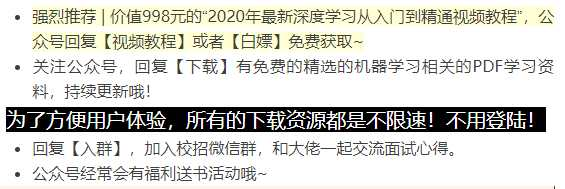# 通俗易懂 | 拉格朗日乘子法

【Nginx】如何实现Nginx的高可用负载均衡？看完我也会了！！

# 从零理解

$$x^2y=3$$# 抽象成数学的形式

$$\min {x^2+y^2}$$
$$s.t. x^2y=3$$

$$\min F(x,y)={x^2+y^2+\lambda(x^2y-3)}$$

【为什么可以这样呢？】

$$\frac{\partial F(x,y)}{\partial x}=2x+\lambda 2xy=0$$
$$\frac{\partial F(x,y)}{\partial y}=2y+\lambda x^2=0$$# KKT条件

• KKT的英文全称：Karush-Kuhn-Tucker

• 可行解在g(x)<0；
• 可行解在g(x)=0。【g(x)<0的情况】

$$\Delta f(x)=0$$

【g(x)=0的情况】

$$\Delta f(x) = -\lambda \Delta g(x)$$

• $$\lambda >=0$$
• $$\lambda g(x^*)=0$$
• $g(x^*) <= 0$